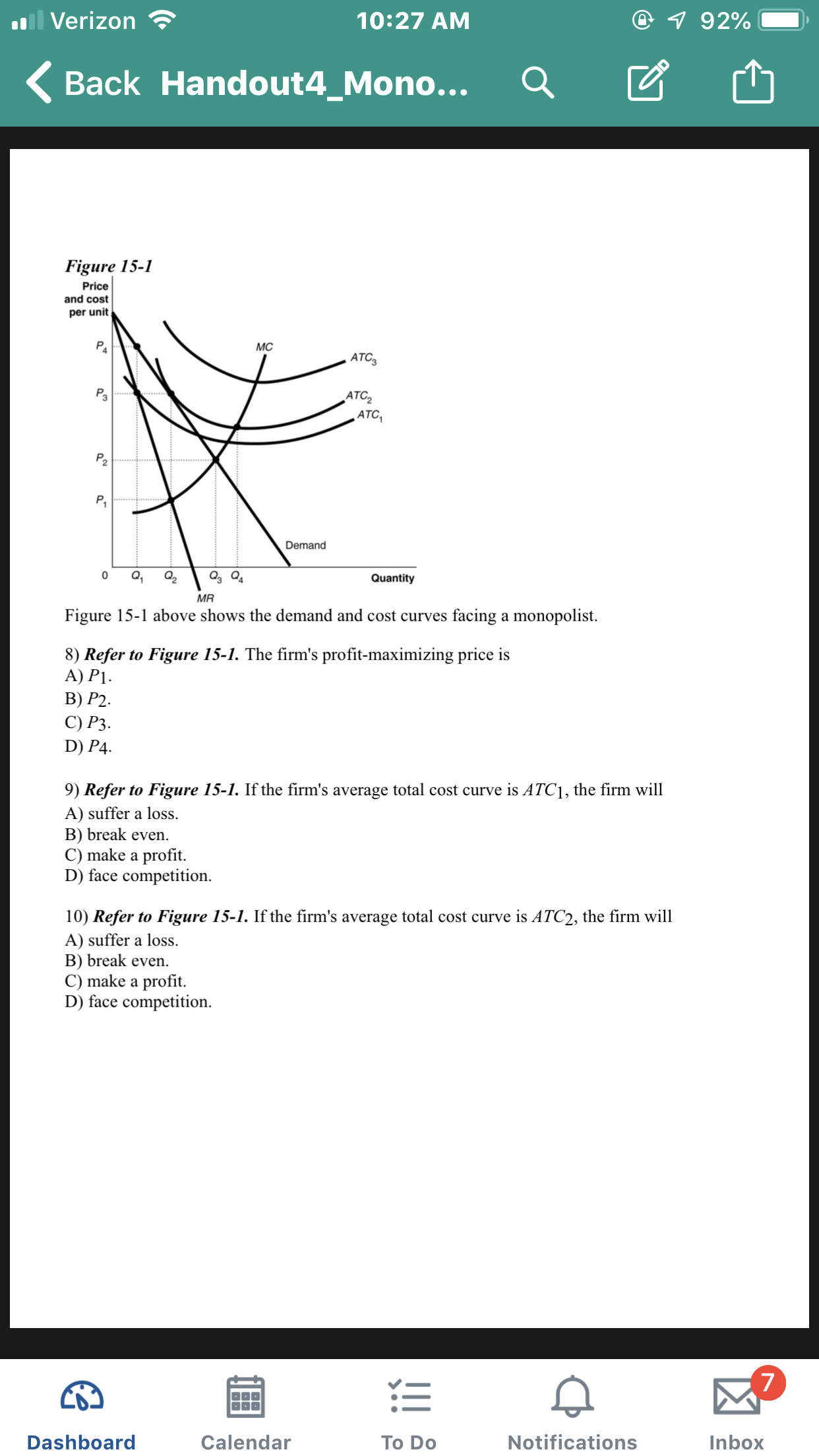il Verizon7 92%10:27 AMBack Handout4_Mono...Figure 15-1Priceand costper unitМCATC3PaАTC,ATCPP.DemandQ2Q40QuantityMRfacing a monopolistFigure 15-1 above shows the demand and cost curves8) Refer to Figure 15-1. The firm's profit-maximizing price isА) P1.В) P2.С) Р3.D) P4is ATC1, the firm will9) Refer to Figure 15-1. If the firm's average total cost curveA) suffer a lossB) break even.C) make a profitD) face competition10) Refer to Figure 15-1. If the firm's average total cost curve is ATC2, the firm willA) suffer a loss.B) break evenC) make a profit.D) face competition7NotificationsDashboardCalendarInboxTo Do

Question

How would you solve thesehelp_outlineImage Transcriptioncloseil Verizon 7 92% 10:27 AM Back Handout4_Mono... Figure 15-1 Price and cost per unit МC ATC3 Pa АTC, ATC P P. Demand Q2 Q4 0 Quantity MR facing a monopolist Figure 15-1 above shows the demand and cost curves 8) Refer to Figure 15-1. The firm's profit-maximizing price is А) P1. В) P2. С) Р3. D) P4 is ATC1, the firm will 9) Refer to Figure 15-1. If the firm's average total cost curve A) suffer a loss B) break even. C) make a profit D) face competition 10) Refer to Figure 15-1. If the firm's average total cost curve is ATC2, the firm will A) suffer a loss. B) break even C) make a profit. D) face competition 7 Notifications Dashboard Calendar Inbox To Do fullscreen
Step 1

In the monopoly market, the profit maximization condition is MR = MC; however, the monopolist charge price according to the consumer’s willingness to pay. Thus, in this case, the monopolist’s profit maximization price is P3. Thus, from the aforementioned options, option C is correct.

Step 2

In the monopoly market, the break-even point is determined by the tangency of the demand curve and ATC curve; however, in the above figure at ATC1, the profit-maximizing price (P3) and the p...

Want to see the full answer?

See Solution

Want to see this answer and more?

Our solutions are written by experts, many with advanced degrees, and available 24/7

See Solution
Tagged in